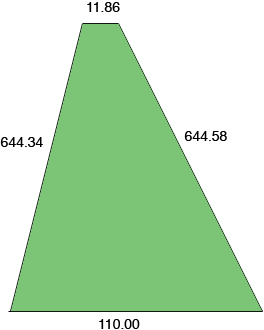SEARCH HOMEMath Central Quandaries & QueriesQuestion from alfredo, a parent: i want to divide my land. It is north is 11.86 ft, the S is 110.00 ft, the W is 644.34 ft the E is 644.58 ft. which = to 1.606 acre. how many feet going from N to S would it be to make 1.5 acreAlfredo,

I am somewhat confused by your question. I drew a rough diagram of your land (not to scale).If the north side and south side are parallel then this is a trapezoid and the area is the average of the lengths of the parallel sides times the distance between the parallel sides. In this case the average of the lengths of the parallel sides is

(110.00 + 11.86)/2 = 60.93 feet.

The distance between the parallel sides is the perpendicular distance which less than the length of either side, so let's say it is 644 ft then the area is

60.93644 = 39239 sq ft.

An acre is 43560 sq ft so this is less than an acre. Even if you take the distance between the parallel sides as 645 ft the area is still less than an acre. I don't see how that area can be 1.606 acres.

PennyMath Central is supported by the University of Regina and The Pacific Institute for the Mathematical Sciences.